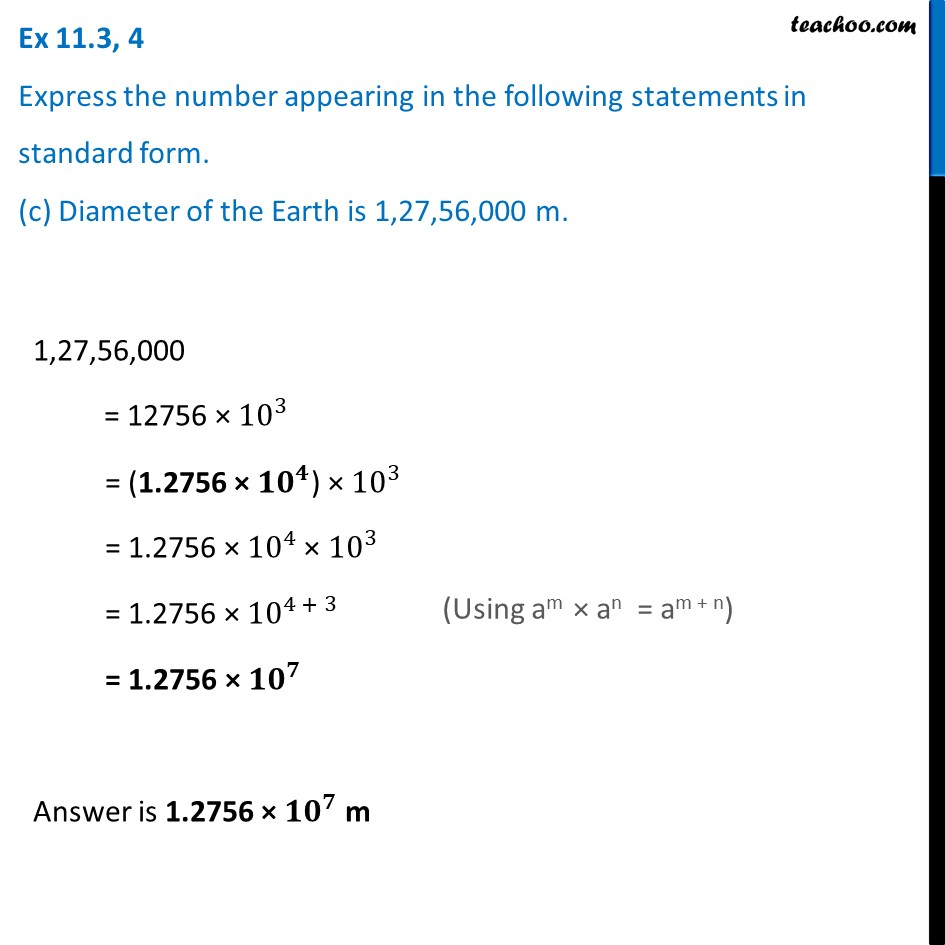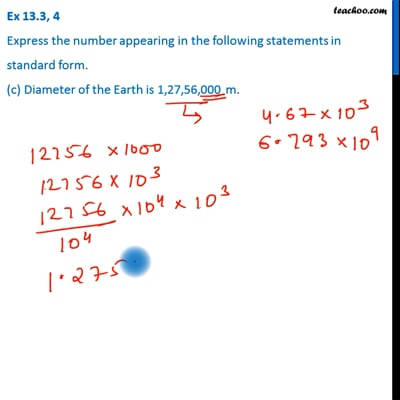Ex 11.3

Chapter 11 Class 7 Exponents and Powers
Serial order wiseThis video is only available for Teachoo black users

Learn in your speed, with individual attention - Teachoo Maths 1-on-1 Class

### Transcript

Ex 11.3, 4 Express the number appearing in the following statements in standard form. (c) Diameter of the Earth is 1,27,56,000 m. 1,27,56,000 = 12756 × 〖10〗^3 = (1.2756 × 〖10〗^4) × 〖10〗^3 = 1.2756 × 〖10〗^4 × 〖10〗^3 = 1.2756 × 〖10〗^(4 + 3) = 1.2756 × 〖10〗^7 Answer is 1.2756 × 〖𝟏𝟎〗^𝟕 m (Using am × an = am + n) Answer is 3.84 × 〖𝟏𝟎〗^𝟖 m (Using am × an = am + n)